By ritz925
• 1900 BCE

# System of Equations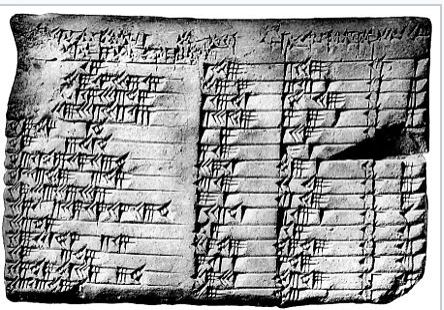Babylonians solve system of equations.
• 1800 BCE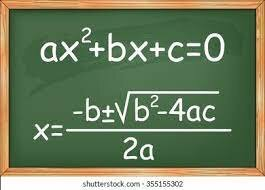• 250 BCE

# Algebraic Equations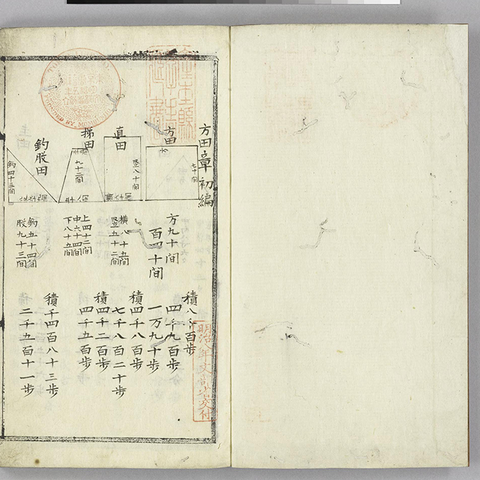Chinese solve algebraic equations.
• 1545

# Cubic Equations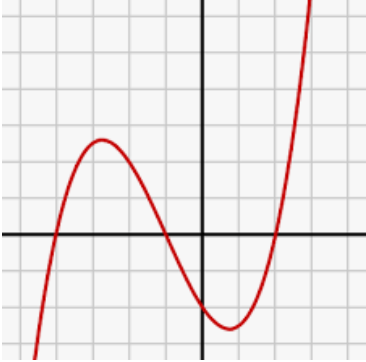Del Ferro was the first to solve the cubic and quartic equations. Multiple roots and complex numbers were introduced for the first time.
• # Step by Step Equations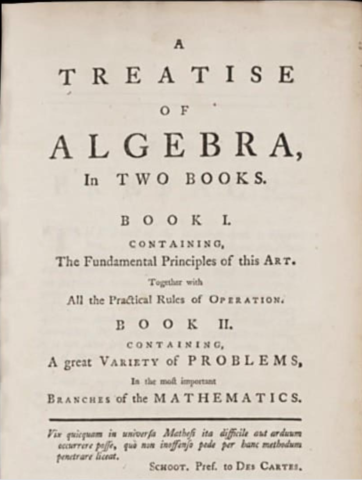The first step by step algebra problems were published in A Treatise of Algebra by William Emerson.